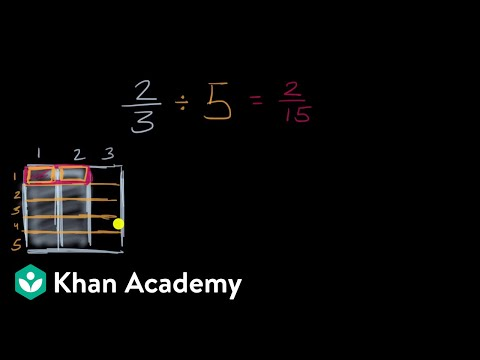# Dividing fractions: 3/5 ÷ 1/2 (Full video)

Description: Dividing two fractions is the same as multiplying the first fraction by the reciprocal of the second fraction. The first step to dividing fractions is to find the reciprocal (reverse the numerator and denominator) of the second fraction. Next, multiply the two numerators. Then, multiply the two denominators. Finally, simplify the fractions if needed. Created by Sal Khan and Monterey Institute for Technology and Education.

### Other videos you might be interested in### Dividing Fractions Word Problems (Full video)

#### Student Achievement Partners